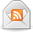# How to Calculate Annual Income

Posted by Don on July 21, 2015

Getting paid is great. Checking your bank account and seeing a higher balance after your pay has been deposited is an awesome feeling and for some people, even a relief. Trying to figure out your annual income, especially when trying to set up a budget, can be tricky if you are paid bi-weekly. How do you calculate annual income? Here are the steps to help you calculate your annual income.

### How to Calculate Annual Income

While on the surface it might seem like a quick and easy task to calculate your annual income, there are some things you have to do before you can even perform the calculation. Luckily, none of these things are very difficult. It is just that everyone’s situation is different and therefore, there is no a one size fits all approach here.

### Factors to Consider When Calculating Annual Income

Gross or Net?

The first thing you have to determine is what your goal is. Do you want to know your gross annual income (your pay before taxes) or do you want to know your net annual income (your pay after taxes)? Once you figure this out, you can take the next steps.

Pay Frequency

The next step you have is to determine how frequently you are paid. Some people are paid weekly, others bi-weekly. Some are paid twice a month and still others only once a month. Before you can calculate annual income, you have to know your pay frequency so you can get an accurate number.

### Do The Math

Once you figure out the two steps above, you can then calculate your annual income. The good news is that it only involves simple math. Let’s look at a few examples.

Gross pay, weekly paycheck

If you are looking to calculate your gross income, and are paid weekly, your first step is to look at your pay stub. On there you will see a breakout of your gross pay and all of the deductions taken from your pay. You will also notice two columns, a “current” and a “year to date” (sometimes listed as “YTD”). Note that sometimes the year to date numbers are the ones near the bottom of the pay stub.

Take the number under the current column heading and simply multiply that number by 52 since there are 52 weeks in the year. Your answer is your gross annual pay.

Net pay, weekly paycheck

Figuring out your net pay is exactly that same as above. The only difference is that instead of taking the gross number under the current column heading, you are taking the net number and multiplying that by 52.

Other Pay Frequencies

Finding your pay amount is the same for all of the other pay frequencies you may encounter. The only difference is the number you multiply your gross/net income by:

• Bi-weekly: if you are paid bi-weekly, take the gross or net income number you want to calculate and multiply by 26 since there are 26 weeks in a year.
• Bi-monthly: same as above, only this time you are multiplying your income by 24 since you are paid 24 times a year.
• Monthly: simply take your income number and multiply by 12.

### Bi-weekly Budgeting Issue

One note that needs to be made is for those who are paid bi-weekly and are using this information to set up a budget. There are 2 months out of the year where you will get 3 paychecks. It is just the way the calendar works. This little wrinkle does complicate budgeting a bit, so you need to be aware of it. When I was paid this way, I would look at the calendar for the year and map out my paychecks. This showed me which 2 months I would be getting the 3rd paycheck.

### Diving Deeper Into Calculating Annual Income

If you want to take this one step further, you can do the same math on your deductions, like 401k contributions and taxes, to see how much you will pay for the year. You can also use this tool from the IRS to determine the correct amount of withholding from your paycheck so that come tax time, you don’t owe a ton of money or get a huge refund either.

### Final Thoughts

Overall, the process to calculate annual income is rather easy. The key though is to figure out which income number you want to see on an annual basis and then know what your pay frequency is. Once you do these 2 things, you can quickly calculate your annual income.

### More Calculations